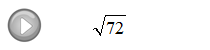## Algebra

### Exponents and Square Roots

To square a number means to multiply that number times itself.  For example:The number 5 is called the base and the integer 2 is called the exponent.

Exponential Notation

Another way to read the above example is "5 raised to the second power."

Simplify.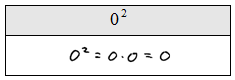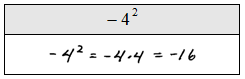It is important to point out the last two solved problems, what is different?

The square root can be thought of as the opposite of squaring a number.  In other words, if we want the square root of 16 the question is "what number squared gives 16?"  Actually there are two answers to this question, 4 and −4 because: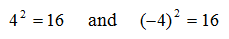Hence, there is a technical distinction here. When we are asked for the square root of numbers we mean the principal (non-negative) square root.

Simplify.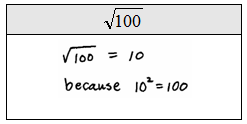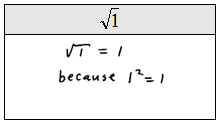If A and B are positive and real the following property is useful for simplifying square roots:Look for the largest perfect square factor and the apply this property as follows: